# Class 11 Maths NCERT Solutions for Chapter 3 Trigonometric Functions Exercise 3.2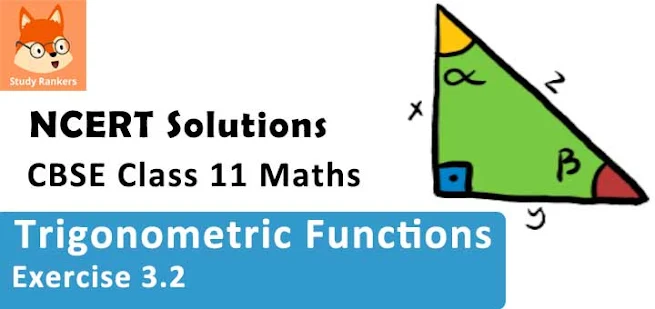### Trigonometric Functions Exercise 3.2 Solutions

1. Find the values of other five trigonometric functions if cos x = -1/2, x lies in third quadrant.

Solution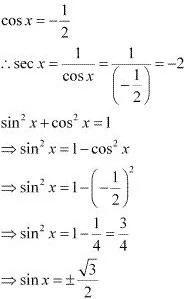Since x lies in the 3rd quadrant, the value of sin x will be negative.
∴ sin x = -√3/2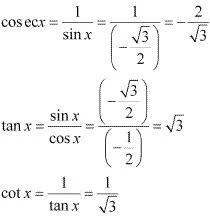2. Find the values of other five trigonometric functions if sin x = 3/5 x lies in quadrant
Solution
sin x = 3/5
cosec x = 1/sin x = 1/(3/5) = 5/3
sin2x + cos2 x = 1
⇒ cos2 x = 1 - sin2 x
⇒ cos2 x = 1 - (3/5)2
⇒ cos2 x = 1 - 9/25
⇒ cos2 x = 16/25
⇒ cos x = ± 4/5
Since x lies in the 2nd quadrant, the value of cos x will be negative
∴ cos x = -4/5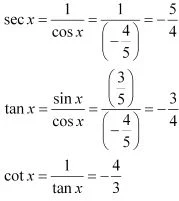3. Find the values of other five trigonometric functions if cot x = 3/4, x lies in third quadrant.
Solution
cot x = 3/4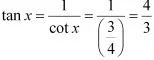1 + tan2 x = sec2 x
⇒ 1 + (4/3)2 = sec2 x
⇒ 1 + 16/9 = sec2 x
⇒ 25/9 = sec2 x
⇒ sec x = ± 5/3
Since x lies in the 3rd quadrant, the value of sec x will be negative.
∴ sec x = -5/3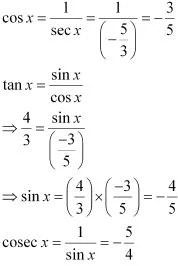4. Find the values of other five trigonometric functions if sec x = 13/5 , x lies in fourth quadrant.
Solution
sec x = 13/5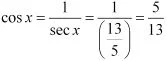sin2x + cos2 x = 1
⇒ sin2 x = 1 - cos2 x
⇒ sin2 x = 1 - (5/13)2
⇒ sin2 x = 1 - 25/169 = 144/169
⇒ sin x = ± 12/13
since x lies in the 4th quadrant, the value of sin x will be negative.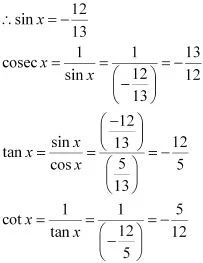5. Find the values of other five trigonometric functions if tan x = -5/12, x lies in second quadrant.
Solution
tan x = -5/12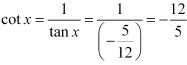1 + tan2 x = sec2 x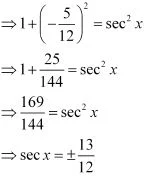Since x lies in the 2nd quadrant, the value of sec x will be negative.
∴ sec x = -13/12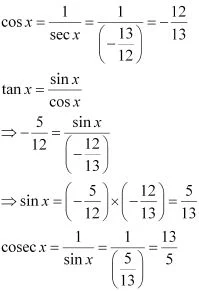6. find the value of the trigonometric function sin 765°.
Solution
It is known that the values of sin repeat after an interval of 2π or 360°.
∴ sin 765° = sin(2×360° + 45°) = sin 45° = 1/√2.

7. Find the value of the trigonometric function cosec (-1410°)

It is known that the values of cosec x repeat after an interval of 2π or 360° .
∴ cosec (-1410°) = cosec (-1410° + 4×360°)
= cosec (-1410° + 1440°)
= cosec 30° = 2

8. Find the value of the trigonometric function tan(19π/3) .

Solution

It is known that the values of tan x repeat after an interval of π or  180° .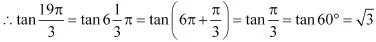9. Find the value of the trigonometric function sin(-11π/3)

Solution

It is known that the values of sin x repeat after an interval of 2π or 360° .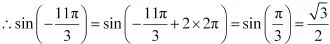10. Find the value of the trigonometric function cot(-15π/4)

Solution

It is known that the values of cot x repeat after an interval of π or  180° .